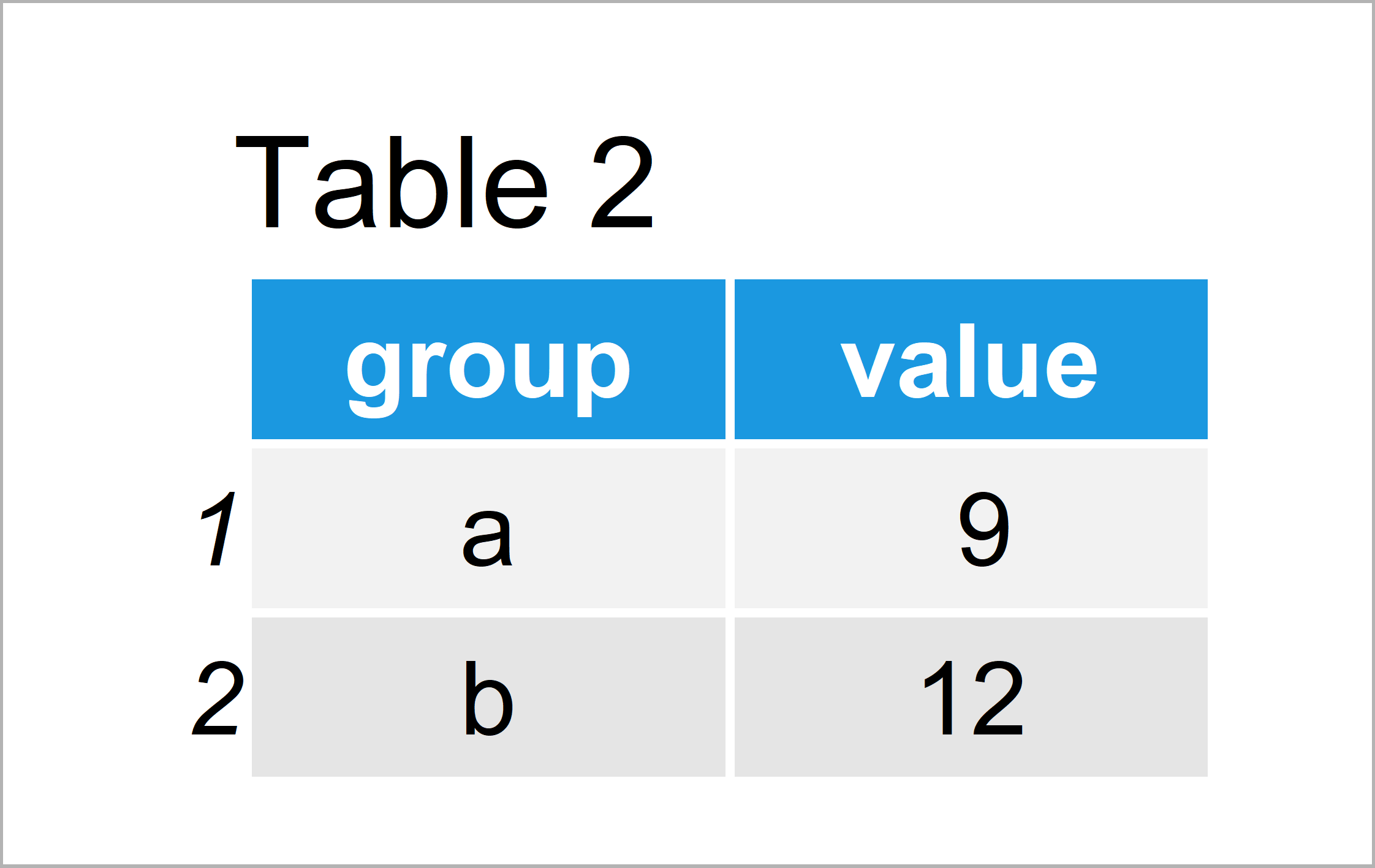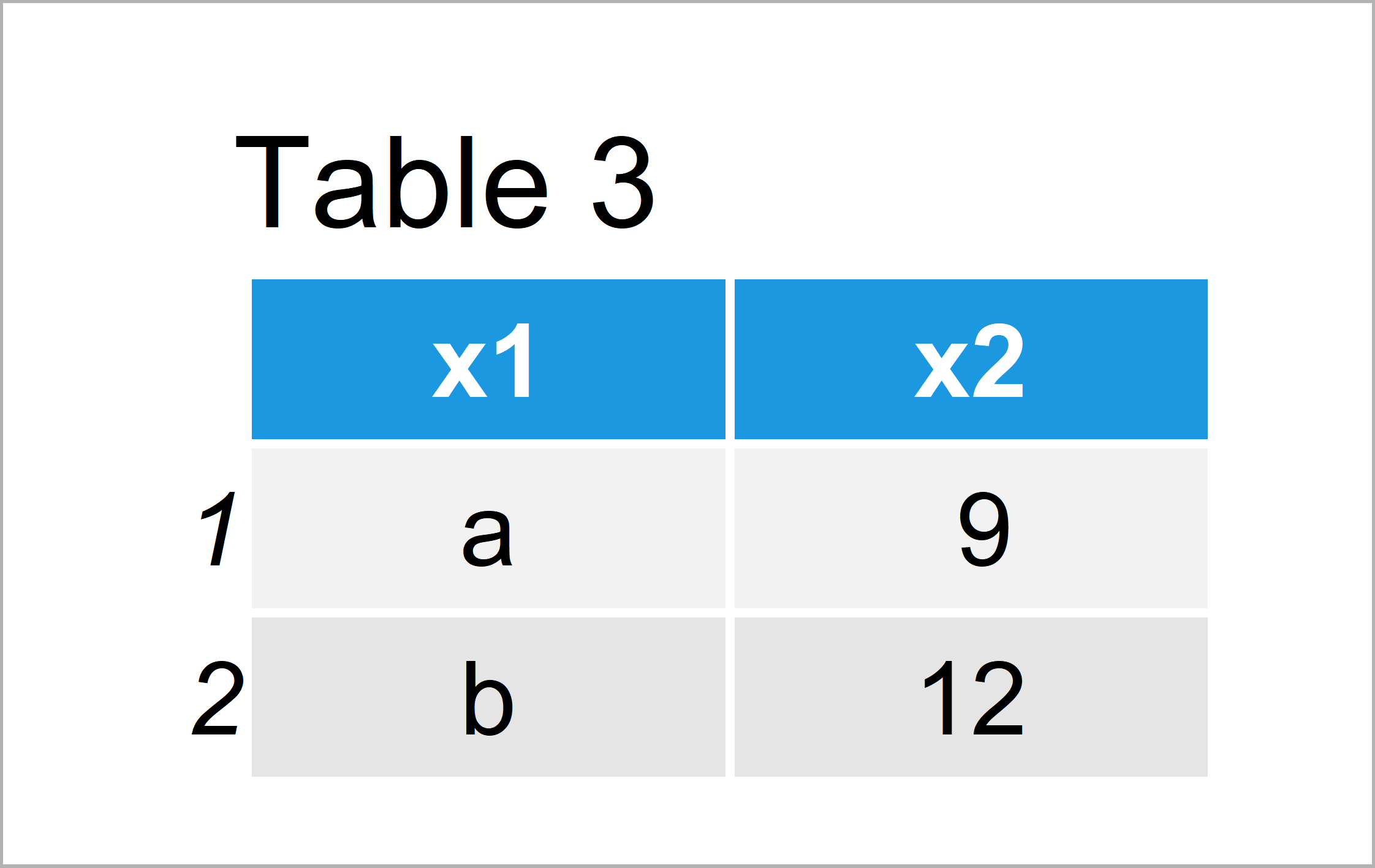# How to Set Column Names within the aggregate Function in R (2 Examples)

This article explains how to change the variable names of an aggregated data frame in the R programming language.

The post contains this content:

Let’s dive right into the examples:

## Creating Example Data

The following data is used as basement for this R tutorial:

```data <- data.frame(value = 1:6,                              # Create example data
group = letters[1:2])
data                                                         # Print example data```As you can see based on Table 1, our example data is a data frame consisting of six rows and two columns.

```data_default <- aggregate(value ~ group, data, sum)          # Default names
data_default                                                 # Print aggregated data```In Table 2 it is shown that we have created an aggregated version of our input data frame. The column names of this aggregated data frame correspond to the column names of the input data frame.

Next, I’ll explain how to change these column names within the aggregate function.

## Example 1: Change Column Names of Aggregated Data Using setNames() Function

The R syntax below illustrates how to use the setNames function in combination with the aggregate function to change the column names of our data frame.

Have a look at the R syntax below:

```data_name1 <- setNames(aggregate(value ~ group, data, sum),  # setNames function
c("x1", "x2"))
data_name1                                                   # Print aggregated data```As shown in Table 3, the previous code has created an aggregated data frame with renamed column names (i.e. x1 and x2).

## Example 2: Change Column Names of Aggregated Data Using list() Function

In the previous example, I have explained how to use the setNames function to rename data frame variables.

However, this example demonstrates how to use the list function within the aggregate function to define our data frame column names.

```data_name2 <- aggregate(list(x2 = data\$value),               # list function
list(x1 = data\$group),
sum)
data_name2                                                   # Print aggregated data```The output of the previous R programming code is shown in Table 4 – It looks exactly the same as the aggregated data frame of Example 1, but this time we have used the list function instead of the setNames function.

## Video & Further Resources

Have a look at the following video tutorial of my YouTube channel. In the video, I show the topics of this article.

In addition, you may want to read the related tutorials which I have published on this homepage.

You have learned in this article how to set the names of columns within the aggregate function in the R programming language. In case you have any further questions, let me know in the comments below.

Subscribe to the Statistics Globe Newsletter